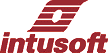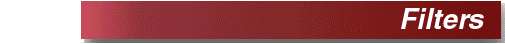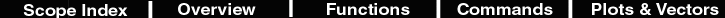Filter <num>
 Description Filters the current waverform using a triangular weight filter +- points wide. The triangle collapses at the endpoints. Hot Key none Script filter current <2*num> Example Open scope and paste this script into the command window. Then press to plot a square wave Select the Calculator menu "Functions//Filters/Filter 50 to check it out. numpoints = 500 time = (vector(numpoints)/(numpoints-1)*1m) newplot pulseplot time setplot pulseplot setunits time sec y = 2*pulse(500u) - 1 plot y